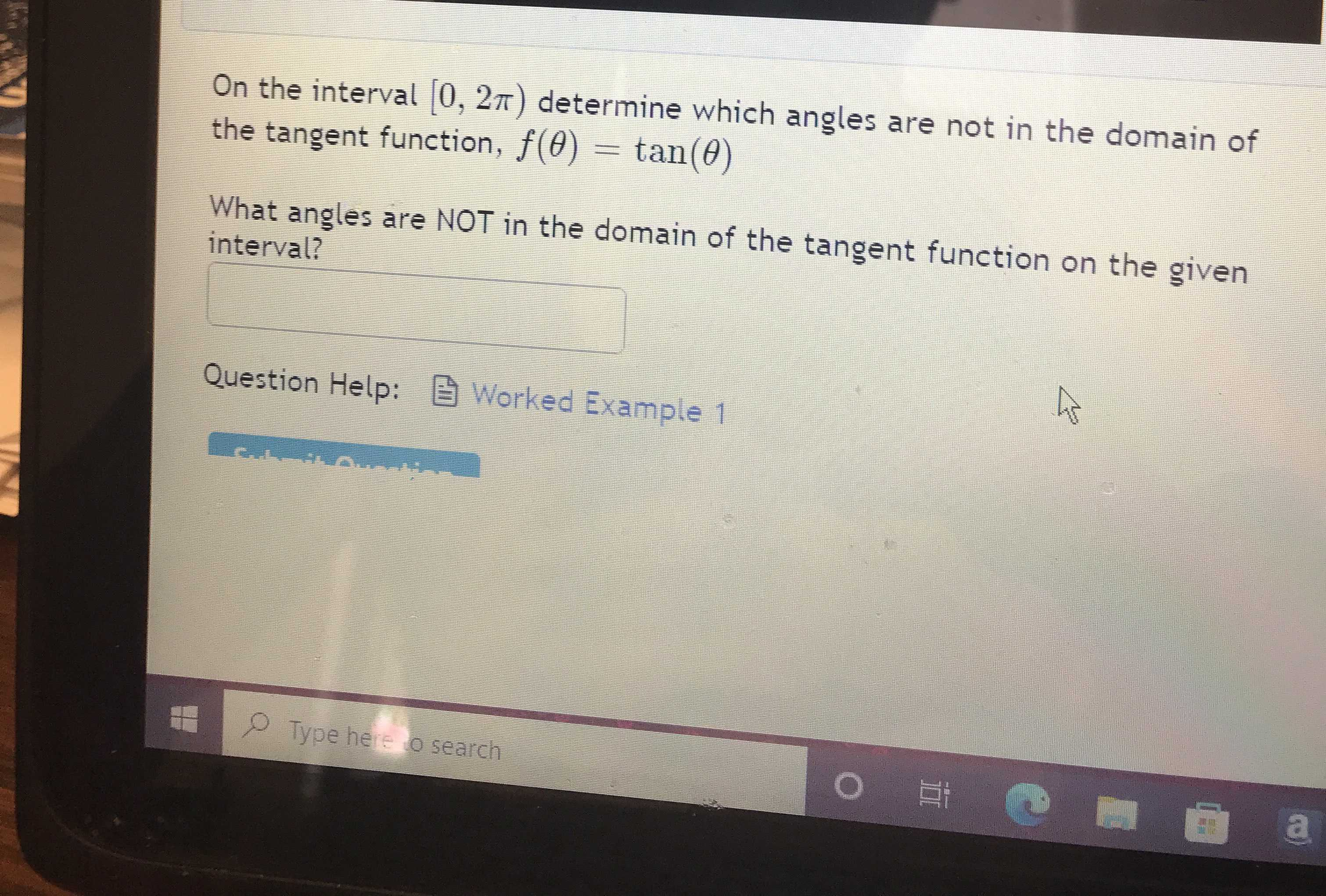### Still have math questions?

Trigonometry
QuestionOn the interval $$[ 0,2 \pi )$$ determine which angles are not in the domain of the tangent function, $$f ( \theta ) = \tan ( \theta )$$

What angles are NOT in the domain of the tangent function on the given interval?

$$\pi /2 ,3\pi /2$$